Photo by Annie Spratt on Unsplash

# The Beautiful Mathematics Behind a Rainbow

Have you ever seen a rainbow? Until recently, I had looked at many rainbows, but had never truly seen a rainbow.

In his writings on faith and logic, religious scholar Bediuzzaman Said Nursi maintained that looking and seeing are two different things. In his book, Words, he says; “The eye is a window through which the spirit looks at this world. If you use it on behalf of your carnal soul, without selling it to God Almighty, by gazing at transient, impermanent beauties and spectacles, it panders to lust and other carnal desires. “

Using Nursi’s reasoning, you might look at a magician who is right in front of you, for example, but never see how a trick is accomplished. The same thing happens when you look at a mathematical equation, but don’t see what the x value is (unless you are good at mathematics).

After watching MIT physics professor Walter Lewin’s lecture about a rainbow, I am finally able to see the rainbow, instead of just looking at it. To share my fascination on this topic, and reinforce my own knowledge, here are some notes I took during Lewin’s lecture.

Walter Lewin is the author of “For the Love of Physics”. This book is really a perfect book to love Physics. You can buy it from here.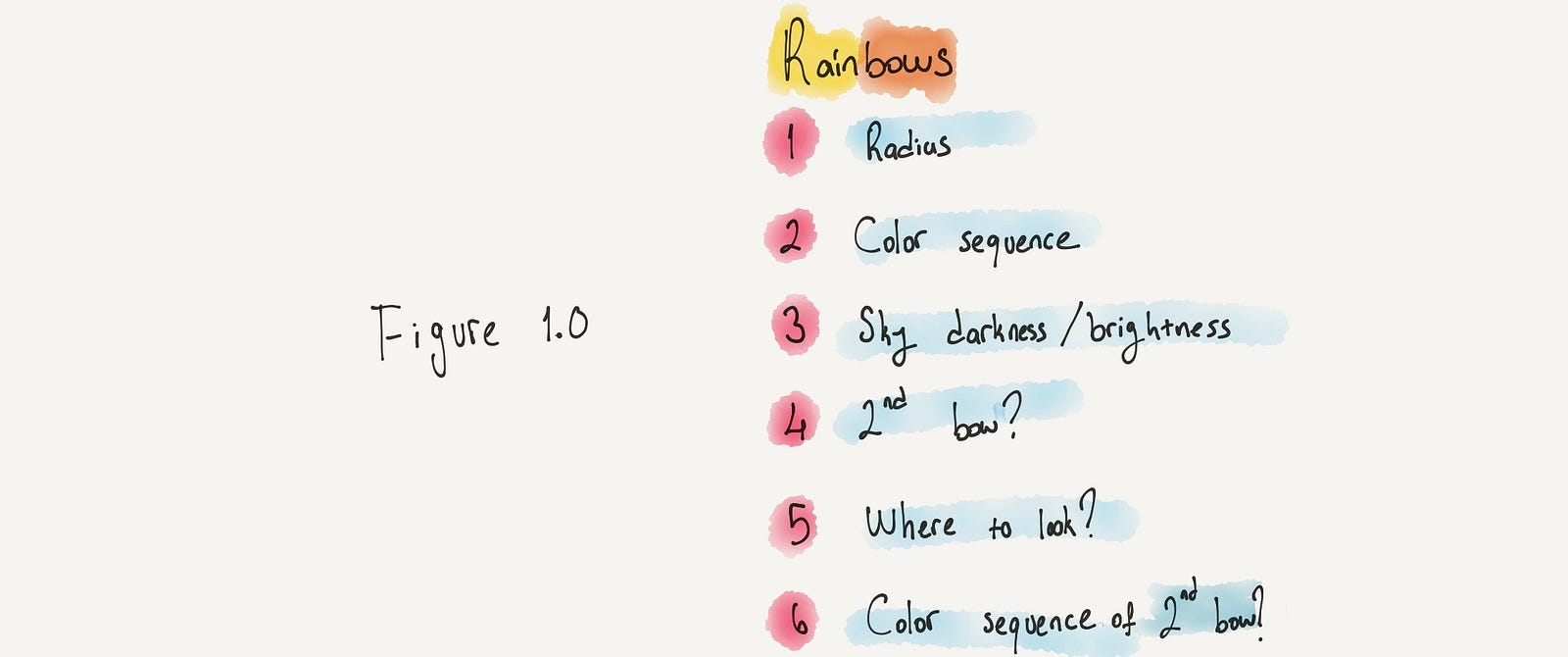Figure 1.0

There are four fundamental facets we need to cover to understand a rainbow well. The first is the “radius” of a rainbow. Since every rainbow has a radius, this means a rainbow is a circle. And every circle has a center point. A rainbow’s center point is below the horizon. Here, we can ask these questions:

1.1 How we can find the radius of a rainbow in degrees?
1.2 Do all rainbows have the same radius?

The second question is about the colors of a rainbow, specifically red. If you see a rainbow, there is always a red color. But:

2.1 Is the color red always on the inside or outside of the rainbow?
2.2 Does the position of the red color depend on the time of the day of and year?

It’s obvious that the light determines a rainbow. If there is a rainbow somewhere, there is an enormous difference in the brightness of the sky above and below a rainbow. We can now ask:

3.1 Where is it bright?
3.2 Where is it dark?

There are some additional questions we need to discuss regarding the second bow, specifically:

4 Have you ever noticed the second bow? If yes, then;
5 Where do you look for the second bow?
6.1 What is the color sequence of the second bow?
6.2 Is it the same as the primary bow, is the brighter, or is it reversed?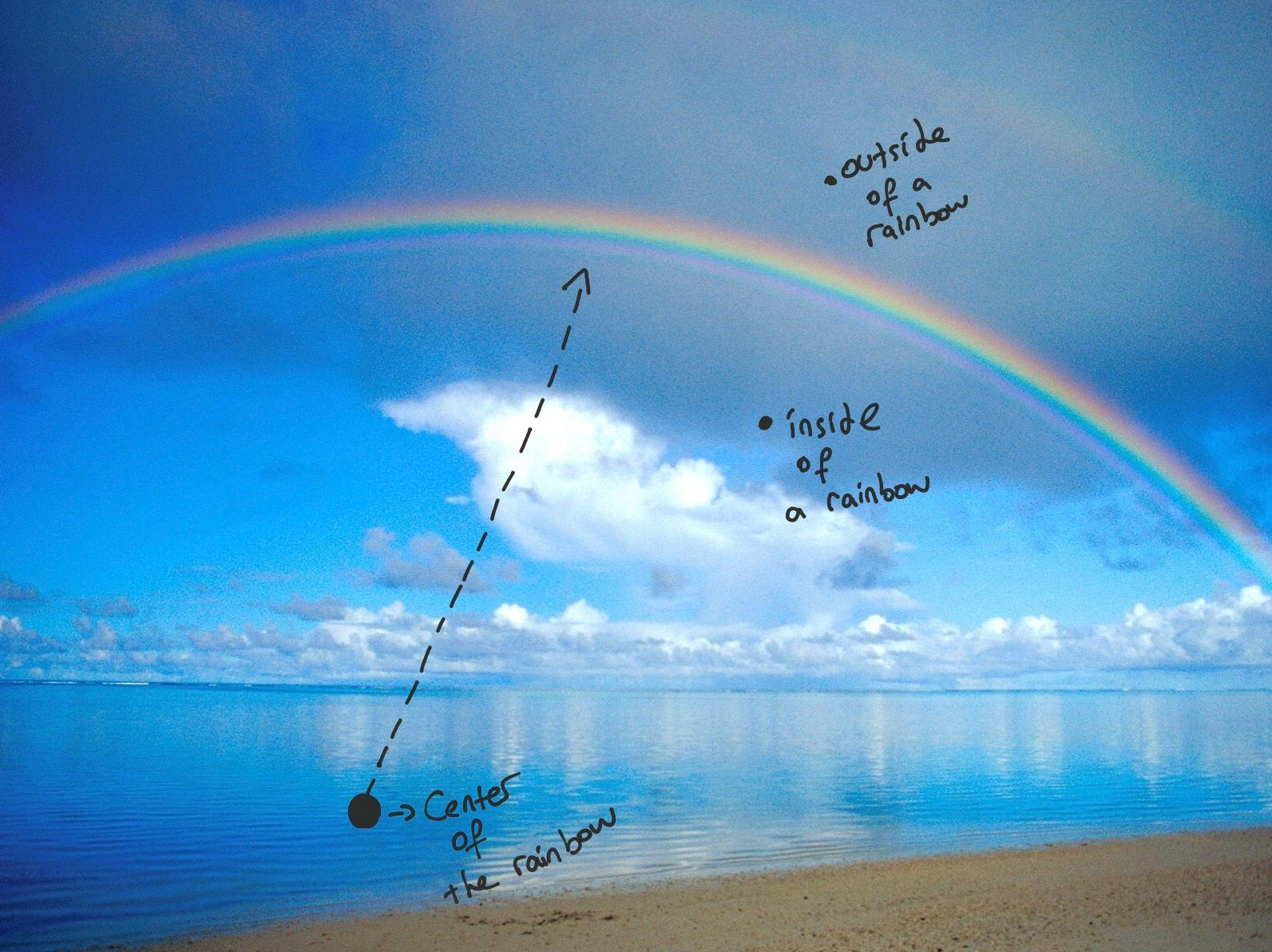Figure 2.0

In order to understand rainbows better, we need to review some basic physics. Let’s start with reflection and refraction.

#### Mediums, Reflection, & Refraction

Definition: A medium is the substance that carries a wave from one location to another.

When light goes from one medium to another, like from air to water, one of two things happens:

• Some of that light bounces back, which we call reflection. The light will reflect at the same angle at which it hit the surface.
• Part of the light doesn’t reflect, instead continuing into water at an angle. We call this refraction.

Each medium has an index of refraction, which tells us what the speed of light in that medium is. The index of refraction of air is 1.0003, and the index of refraction of a vacuum is 1.

That means the speed of light in air and in a vacuum is almost same. That speed is 300,000 kilometers per second, and that’s why the index of refraction for air and vacuum is 1. But in water, the index of refraction is approximately 1.33. The difference between 1 and 1.33 means that in water the speed of light is 33 percent slower than it is in air.

### Snell’s Law and an Angle of Incidence

There is also a connection between angle of reflection, angle of refraction, and the index of refraction. We call it Snell’s law.

Snell’s law illustrated: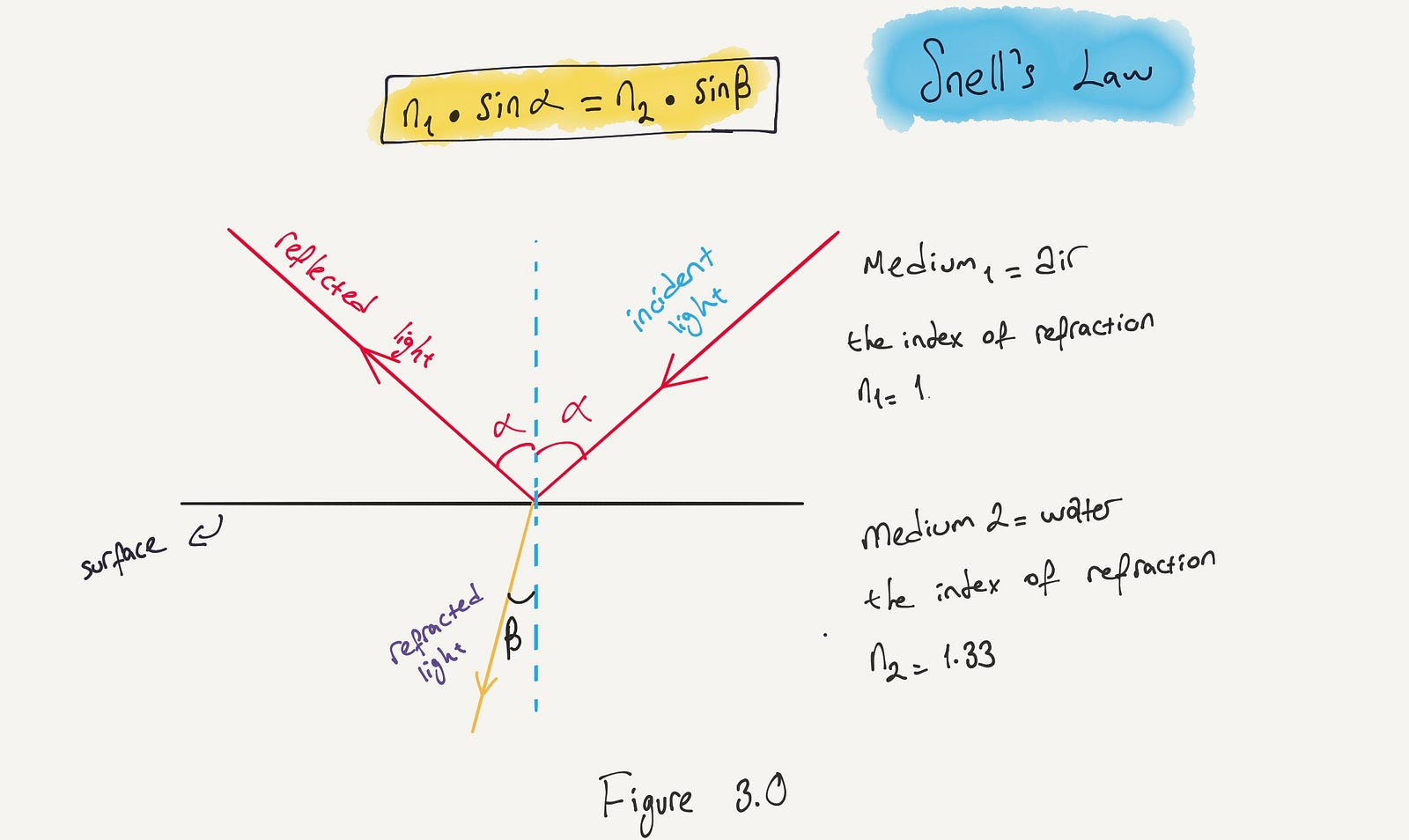Figure 3.0

To understand this formula, you need to know the definition of the sine of an angle.

Definition: The sine is a trigonometric function of an angle. It is found by taking the length of the side opposite the angle and dividing by the length of the longest side of the triangle.
If α is 0°, then sin (α) = 0.
If α is 30°, then sin (α) = 0.5.
If α is 90°, then the sin (α) = 1.

Let’s do an example where we can apply Snell’s law.

Light comes from air to water at an angle of 60°. (This is possible, because the angle at which water comes in, the angle β, will be 40.6°.)

Definition: The angle of incidence is the angle between the incoming light and the vertical on the surface.Figure 4.0

Like professor Lewin said, “very simple, very straightforward.”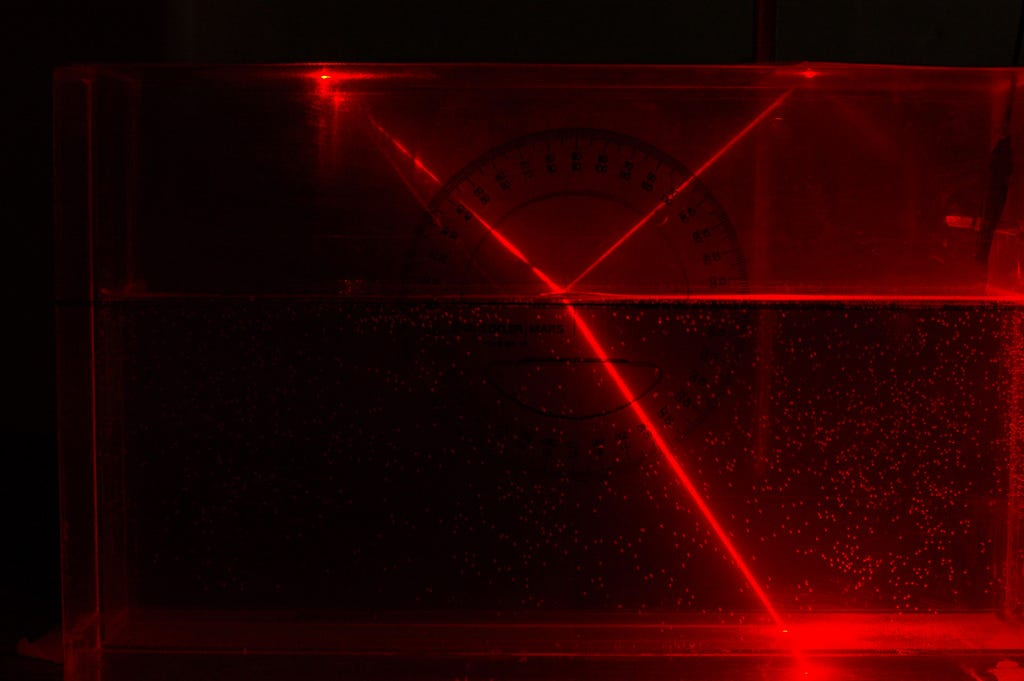Figure 5.0 | You see light coming in from the left side. The angle of incidence α is almost 60°. Some of that light is reflected and some of it going in and that must be then very close to an angle β which is 40°. *5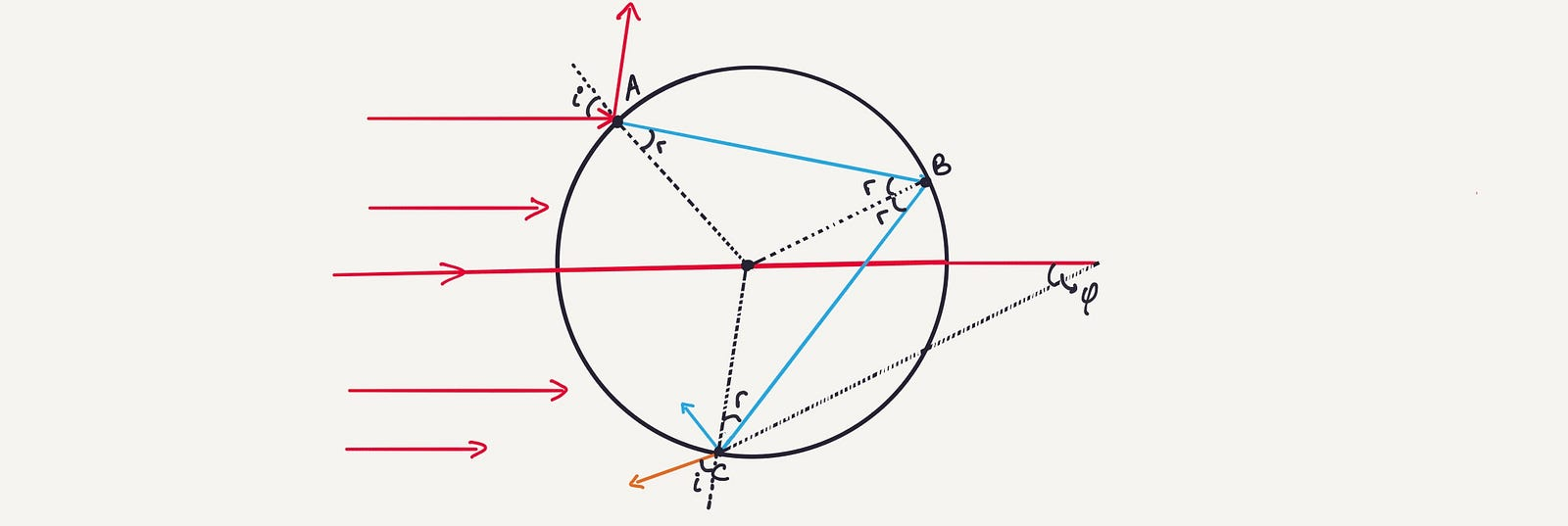Figure 6.0

The figure above displays a true wonder of water. Sunlight is coming from the left side, landing everywhere on half the surface of that raindrop. We can choose one very narrow beam of all that sunlight with a 60° angle of incidence. We call the angle of incidence “i and the angle for the refraction r.”

Of course, that narrow beam is the only a small fraction of the total.

At point A, two things happen (in accordance with Snell’s law);

• A little bit of light reflects.
• The remaining light refracts.

The light reaches point B inside the water drop. At point B, two things happen:

• Some of the light goes back into the air (signaling refraction).
• Some of the light gets reflected. (Therefore, at point B, the angles are same, “r.”)

Then the reflected light goes on its way to point C. Here, a little bit of the light is reflected and goes back into the water. Most of it comes out of the water, which results in refraction.

At this point, if we use Snell’s law at point A, the reflection law at point B, and then Snell’s law at point C again, we will see that our angle for the refraction at point C will be, surprisingly, “i.”

Now, if we check the line which comes out from the water at point C, we will have a changing angle. We can call it φ (Phi). And for the angle φ, we have an algebraic formula, as follows:

φ= 4r — 2i

If you check the figure, you will see that we have 4 r and 2 i.

By the way, If we take the narrow beam which passes through the center of the raindrop, we can easily see that the angle of incidence is zero. In accordance to Snell’s law, this narrow beam goes straight through. Then it reflects back and finally it comes out of water. This is true because:

if i = 0, then r = 0 and therefore φ = 0.

Let’s do something interesting and change the value of “i.” If the light hits the water drop higher up, the angle “i increases. At some point, the angle φ also increases and reaches a maximum value.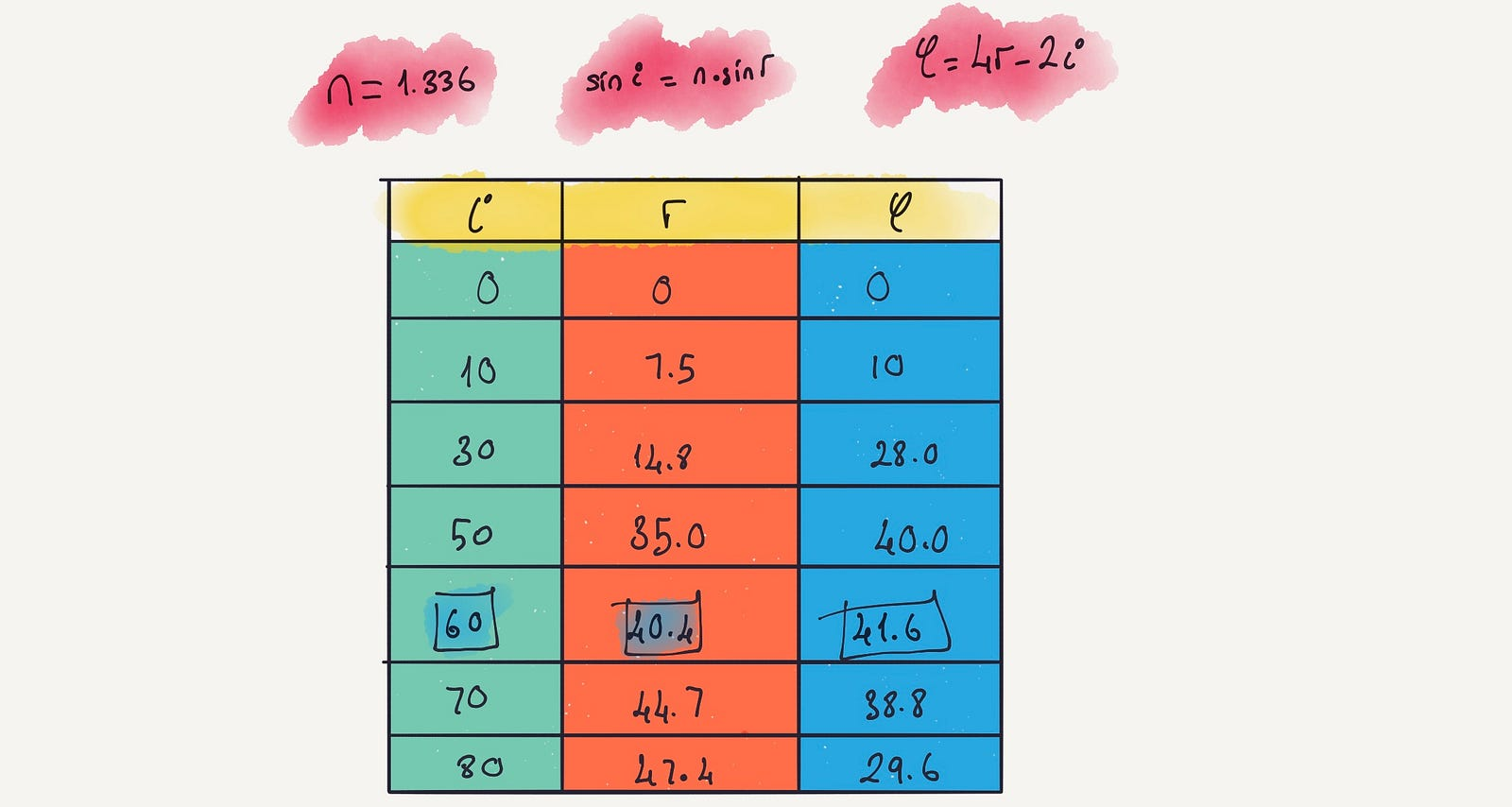Figure 7.0

If you recall, the index of refraction for water is 1.336. If we take any value of “i on the table and apply Snell’s law, we can easily calculate values of r. And then, if we use the formula φ=4r — 2i, we can come up with the values of φ. This is illustrated in Figure 7.0.

If this doesn’t seem all that special, there is something else that you wouldn’t expect: φ reaches a maximum value, and when you go to higher values of i, then φ goes down again. This plays a key role in the formation of a rainbow.

The index of refraction depends on the color of the light.Figure 8.0

As seen on the table, when the angle of incidence for red light is very close to 60°, the value of φ is at its maximum, which is 42.3°. In other words, the value of φ can be smaller than 42.3°, but it cannot be larger. For blue light, the angle of incidence is slightly lower, and the φ maximum is at 40.7°.

### Water Drop & A Cone

A key question arises: Let us say we have one water drop, and the sunlight falling onto that water drop is coming from the left. What is that one water drop going to do with the white light from the sun that covers the half of the rainbow?

Let us check first for red light, and then for blue light.

Light beams will come out of this drop, and that cone will be filled with red light. But the angle for the light beams cannot be larger than 42°, meaning they can be smaller. Then we will see a cone of lights.

But why is it a cone? Because, if we go back to what was previously discussed, we will remember that one light beam comes in with an angle i, and then comes out with an angle i.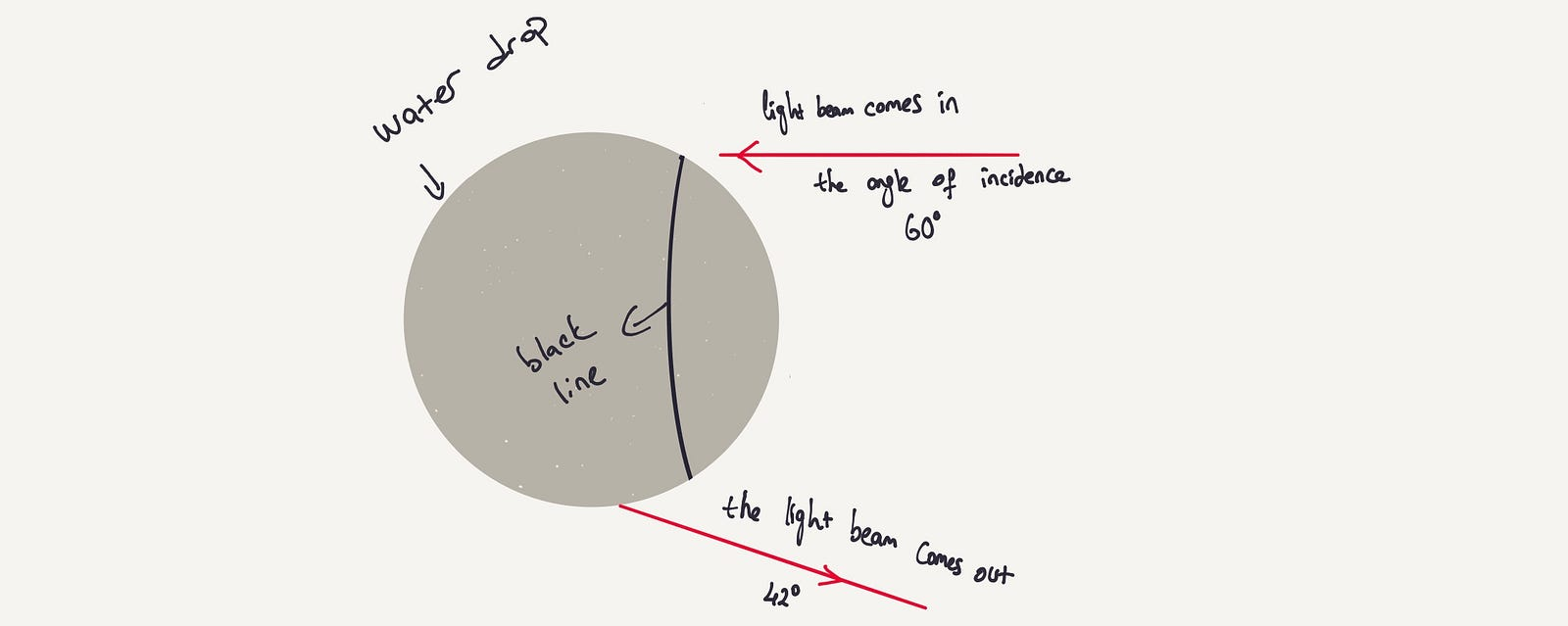Figure 9.0

Figure 9.0 shows a representation of a water drop. The light that comes in at the angle of incidence is 60°. It refracts and reflects inside the droplet, then goes to point C and comes out of droplet at an angle of 42°. And, surprisingly, if the angle of incidence is 60°, when the light beam comes out, it has to be red light. On the other hand, there is not only one light beam comes in with an angle 60°. The entire surface of this water drop is illuminated by the sun, so there’s an enormous number of beams for which the angle of incidence is 60°.

If we go back to Figure 9.0, we see the black line on this water drop. Any light beam that comes in anywhere on that black line will have an angle of incidence of 60°.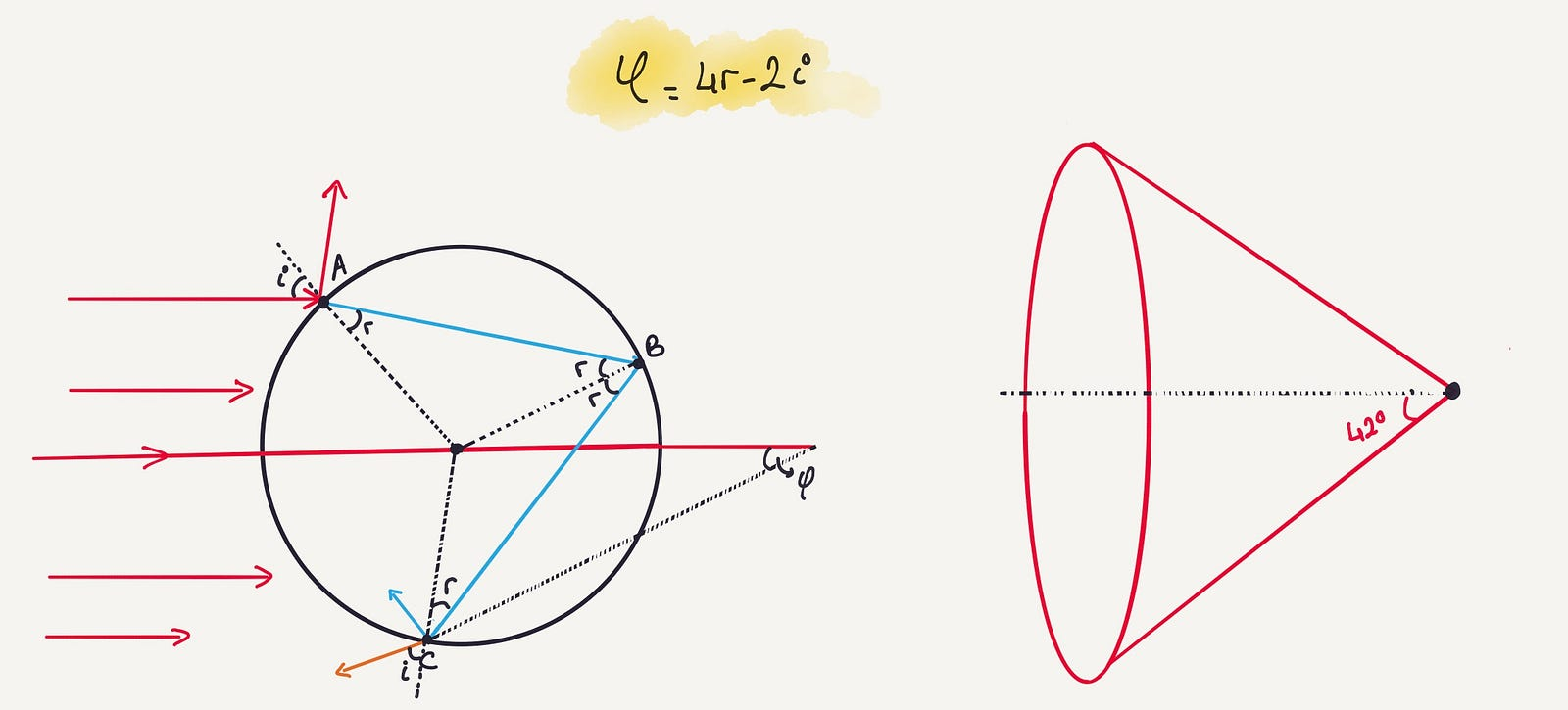Figure 6.1

Now we will do the same for blue light. This time, we will have another cone which is completely filled with blue light, but when the blue light beams come out, the angle will be at a maximum of 40.7°.

Interesting fact: All the colors except for red and blue that are in the sunlight will be in between the red and blue.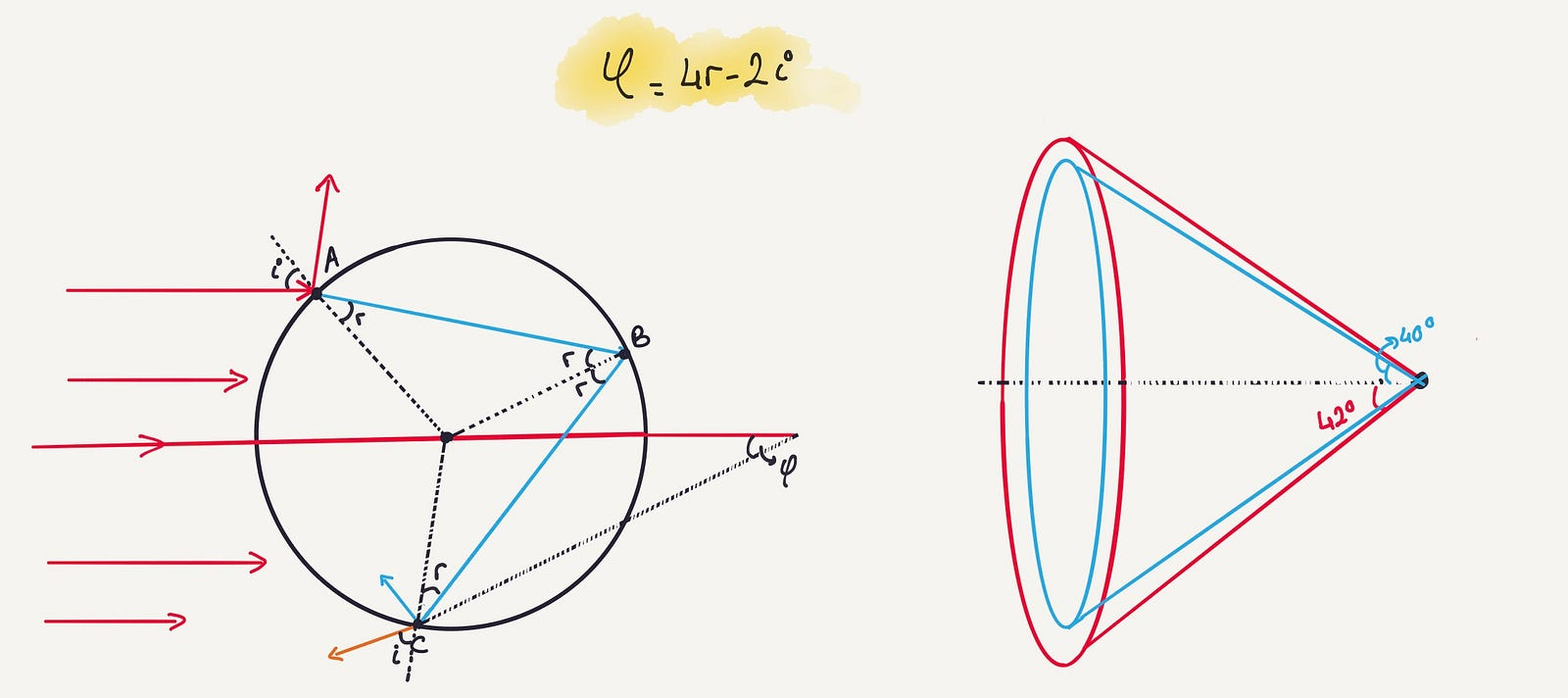Figure 6.2

### A Journey: Refraction, Reflection, and Refraction

We know that when the light comes in, it goes through a journey. A light beam refracts, then reflects, and then refracts. That’s all. But, how about the light on the right side of the cone? Is there any light? When the sun shines in, does this water drop bring light there?

If the angle φ is larger than 42°, then, yes, it is possible. But we have already proven that the angle φ cannot be larger than 42°. So it is impossible: There is no light outside the cone.

Another question… What will be the color of the light inside the blue cone? We should remember that the red light is everywhere in this cone, because as long as the angle φ is smaller than 42°, red light can be anywhere. The blue light is also almost everywhere because the blue light is allowed to be smaller than 40°. This means all the colors that we forgot are coming out inside the blue cone and there will be just white light.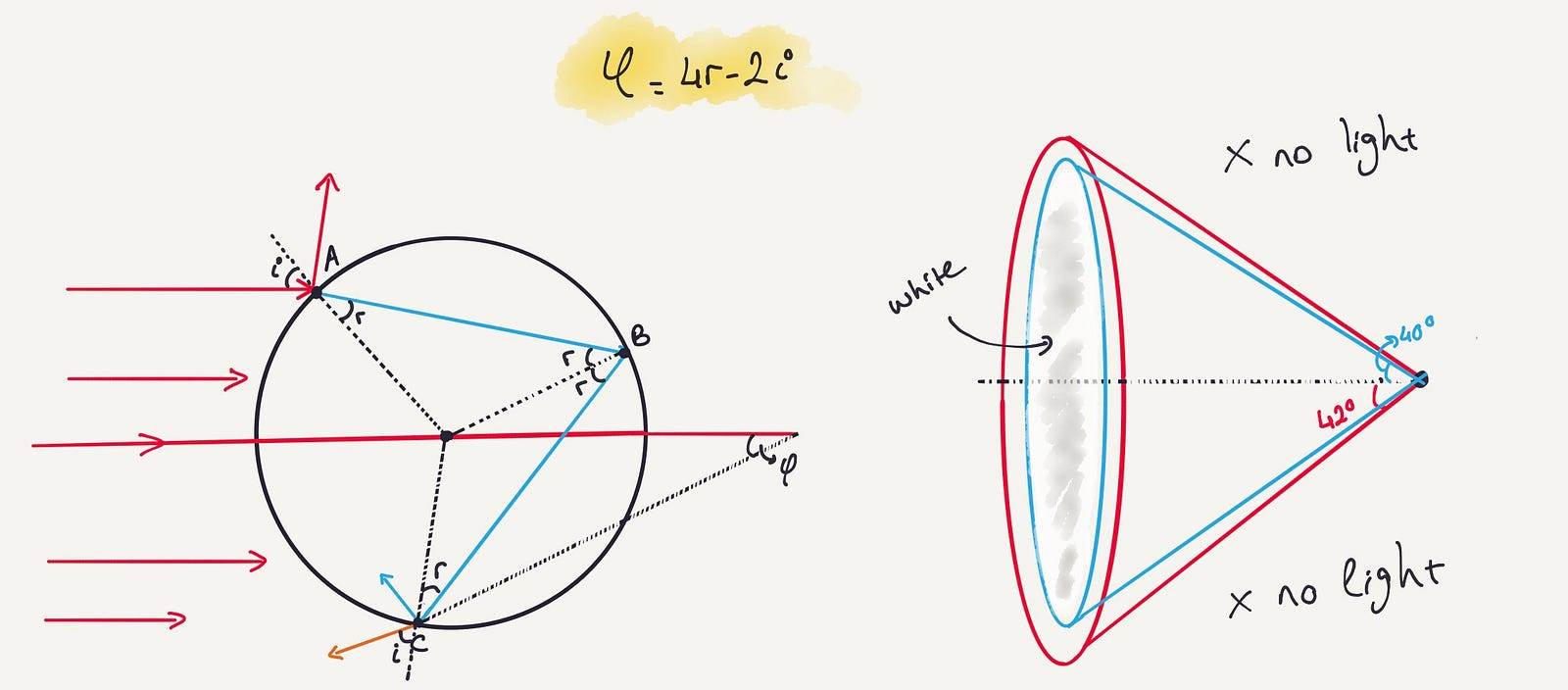Figure 6.3

To summarize, if we have a water drop, and a bright light shining onto this water drop, we will see red, blue, green, white, and other colors inside the cone or circle. In other words, all the colors of a rainbow. And of course, there will be no light outside the cone. There are some experiments on YouTube that you can watch if you want.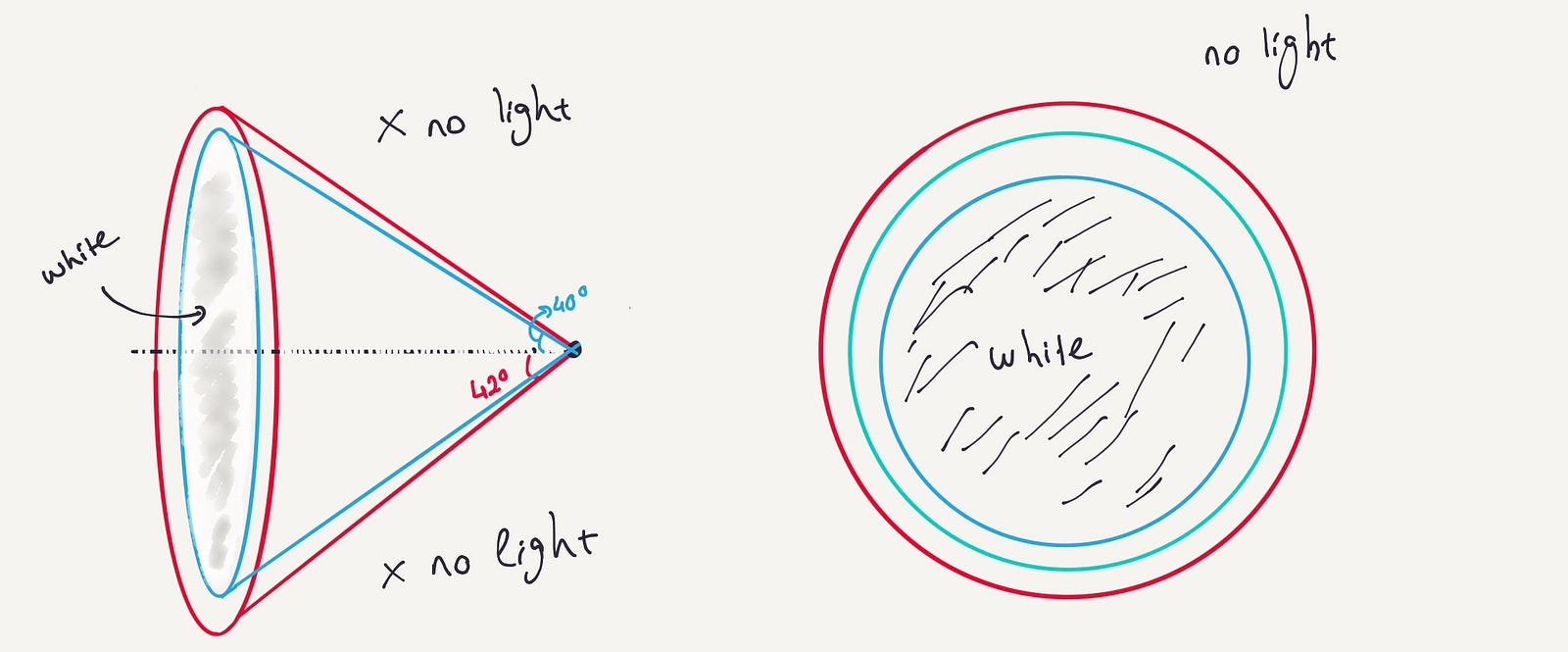Figure 6.4

You may think that at the location where blue comes out, if all the other colors can come out — which they can because the angle is smaller than 40° — the light should be also white, not blue. It is a nice approach, but wrong.

It is wrong, because of the light intensity. If you check the graph for the intensity of the light, you will see that different colors have peak points where φ reaches the maximum value. If all the colors are present and equal intensity, we see white light. That’s why the inside of the cone is white. However, when the color red spikes up, it dominates its location. We don’t even notice the other colors.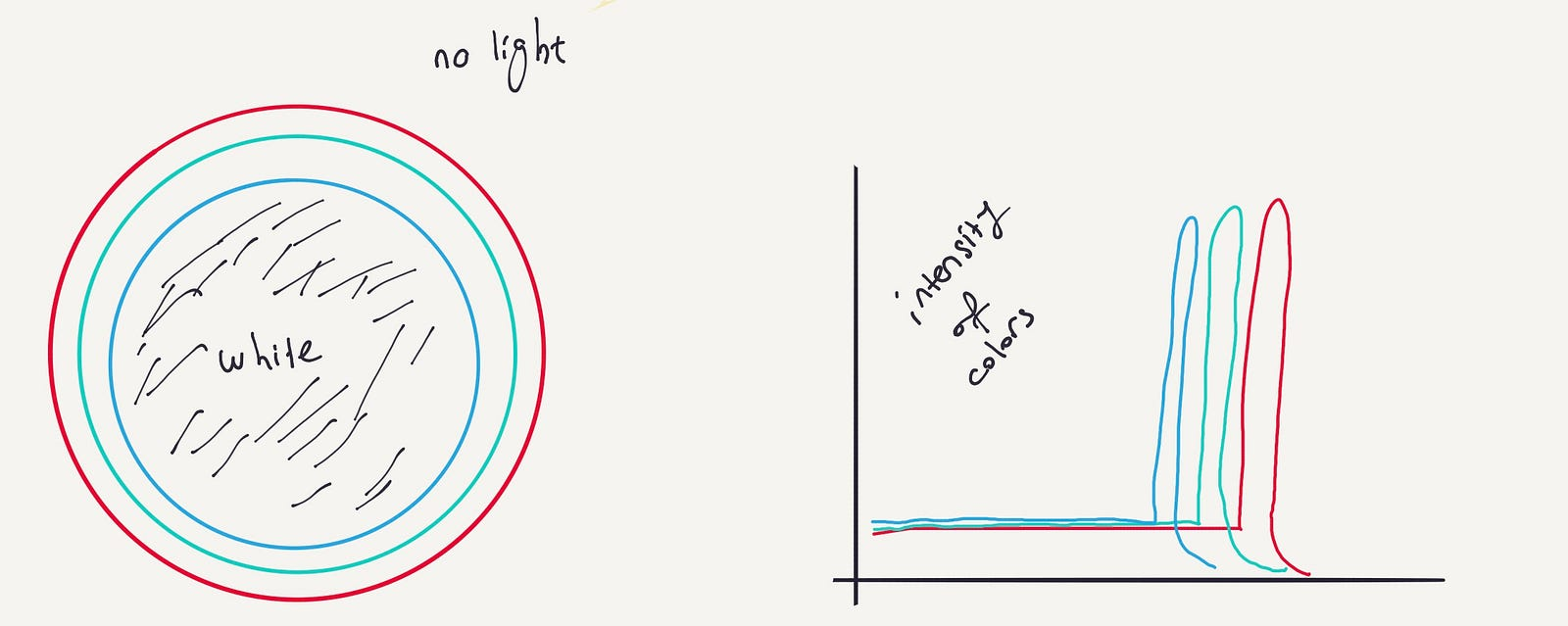Figure 6.5

### Looking at the Sky

Let’s say you are standing at point A and the sunlight is coming from the left. Since the light comes from infinitely far away, all these lines are parallel to each other. Let’s say you look at the sky in direction B and pick a raindrop at any point. Any point you pick will cast the cone in the direction of the sun with the angle 60°.

When you look at the sky in the direction B, will you see light? You will not, because you are outside the 42°.Figure 10.0

What if you look in a different direction? We will look at the point C (it doesn’t matter where), and take a rain drop on line C. Light beams will do the same thing. Again, they will produce a 42° red cone. However, you will see white light. Yes, you read that right, white light, because you are looking straight in the middle at white light.

Let’s say we choose another angle and look at point D. If we choose a raindrop anywhere on line D, it will make no difference. It will cast into the sky the famous 42° angle because that’s the angle that was chosen there. If we look in that direction of the sky, what will we see? Yes, only red. No other color!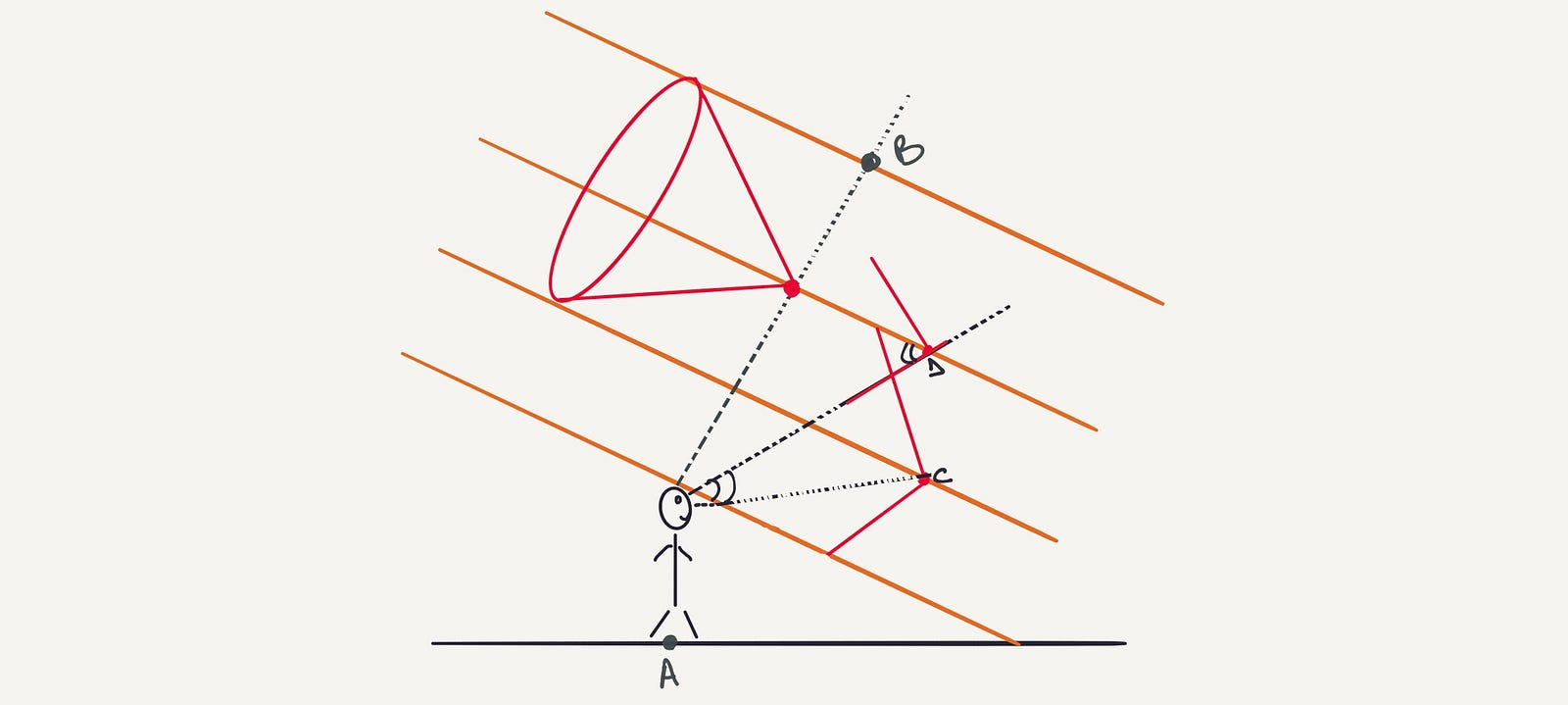Figure 10.1

And now, we know where we will see red light, blue light, or white light. And we also know which direction has no light.

### A Rainbow Is a Bow in the Sky

Let’s suppose the sun is on your left and you are standing at point A (Figure 15.0). Since there is light, you will have a shadow on the ground. As long as you look 42° away from any direction, you will only see red. That is because a cone is a spherical object. And that explains why the rainbow is a bowl in the sky. As long as it is 42° away from a line in any direction, you will always see red light. And if you decrease the angle, you will see the other colors in the sky.

And so now, we can make a picture of a rainbow.

When we look at the horizon, sometimes we see a rainbow. And we will have a center point somewhere below the horizon. The radius with 42° has red light. The blue light is closer to the 40° radius. This tells us that the red bowl is always on the outside and the blue light is always on the inside. That’s what we always see.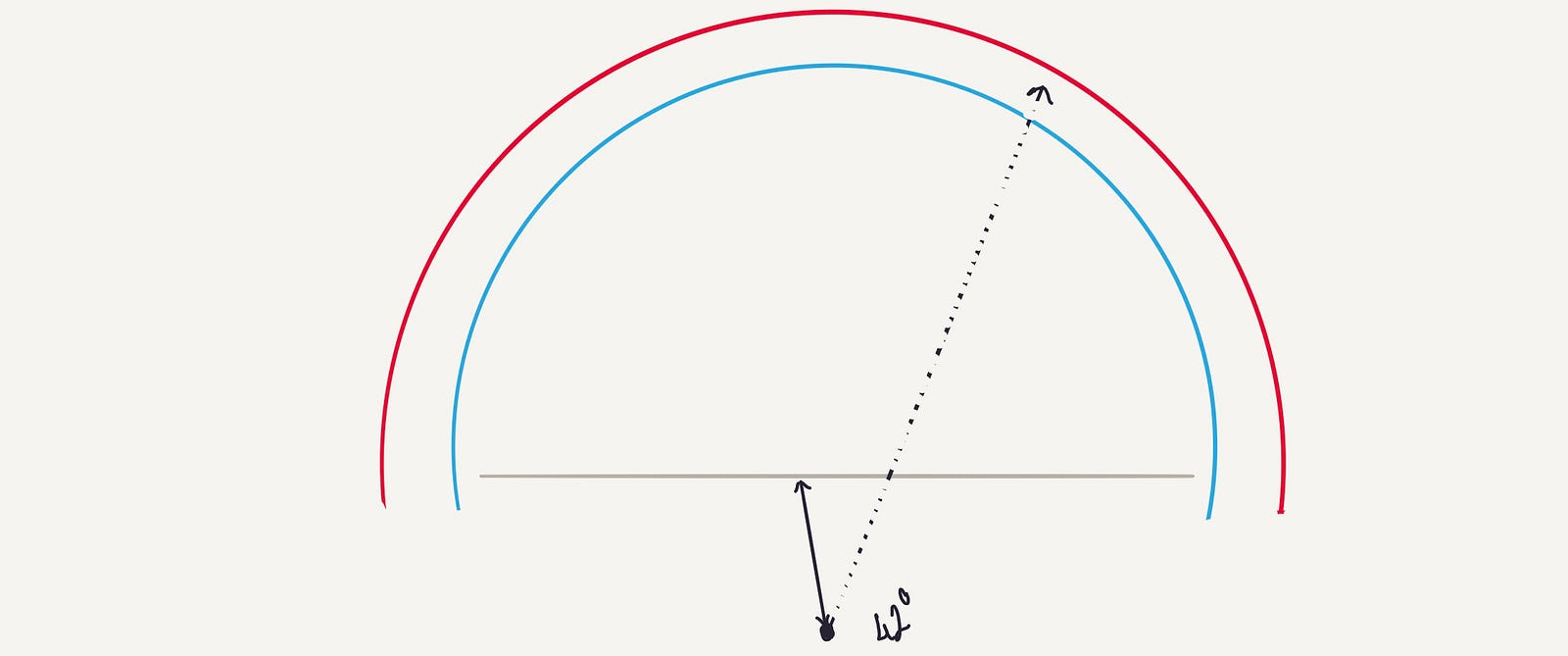Figure 11.0

### Double Reflection in the Rainbow

We can also include two reflections in the rainbow. If we do, we get refraction, reflection, another reflection, and then another refraction. So the light beams reflect twice. And if we do this hocus-pocus again and again, we can come to a conclusion: There is no maximum value for the various colors, but there is a minimum value. In other words, various colors cannot be lower than a certain value, but they can be higher.

And if we do some calculation for the angles, we will see something very similar. We will find out that the second bow is pointing at the sky, and the angle is 10° above the first one (which is 52°). And, surprisingly, the colors of the second bowl reverse. In other words, red is the one on the inside, and blue is on the outside this time.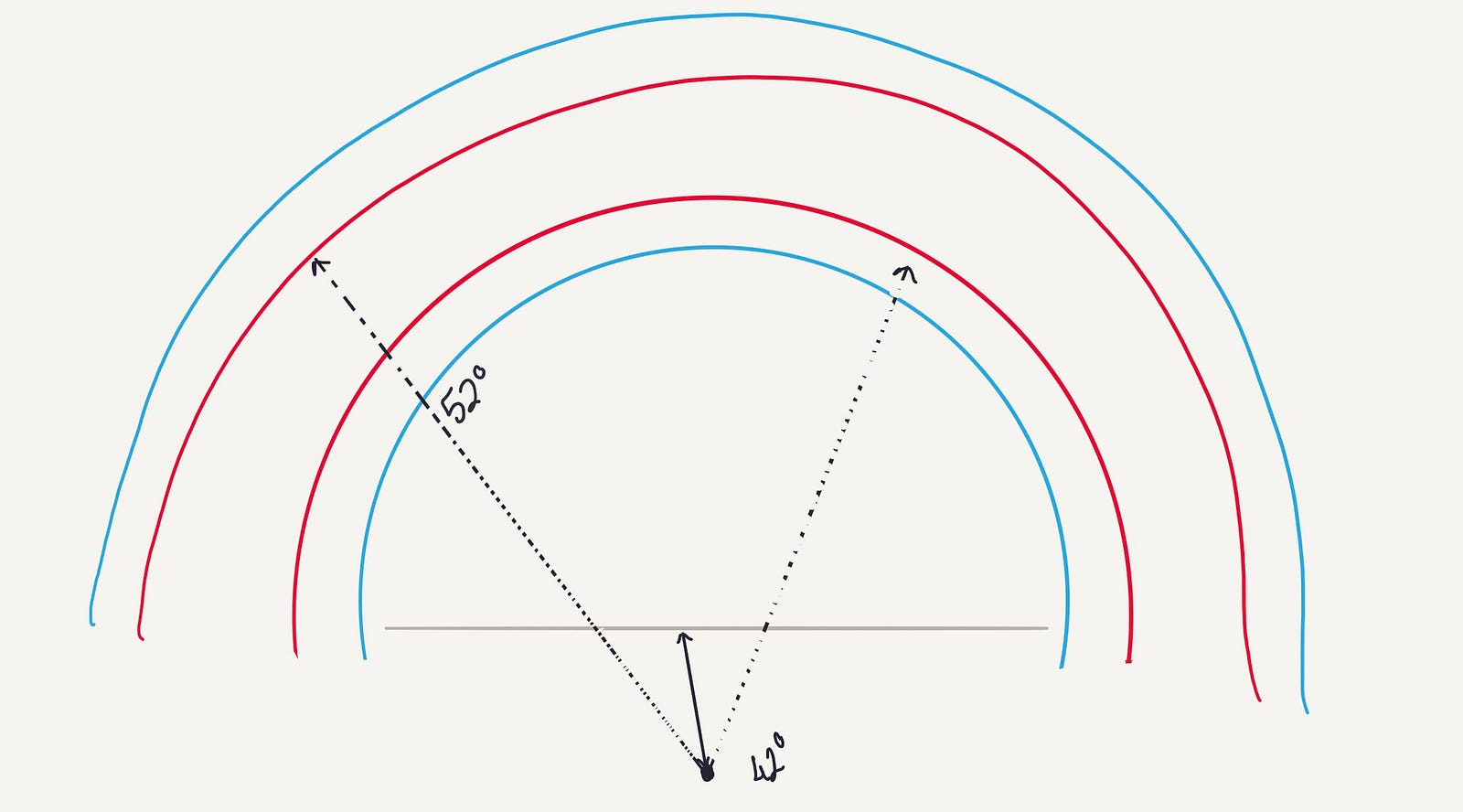Figure 11.1

Interesting fact: There is no light between the two bowls. This is because of the φ maximum and minimum. We call that dark part Alexander’s dark band. Additionally, since the second bowl is formed by φ minimum, sunlight can come out at angle φ or larger and there will be sunlight above a rainbow.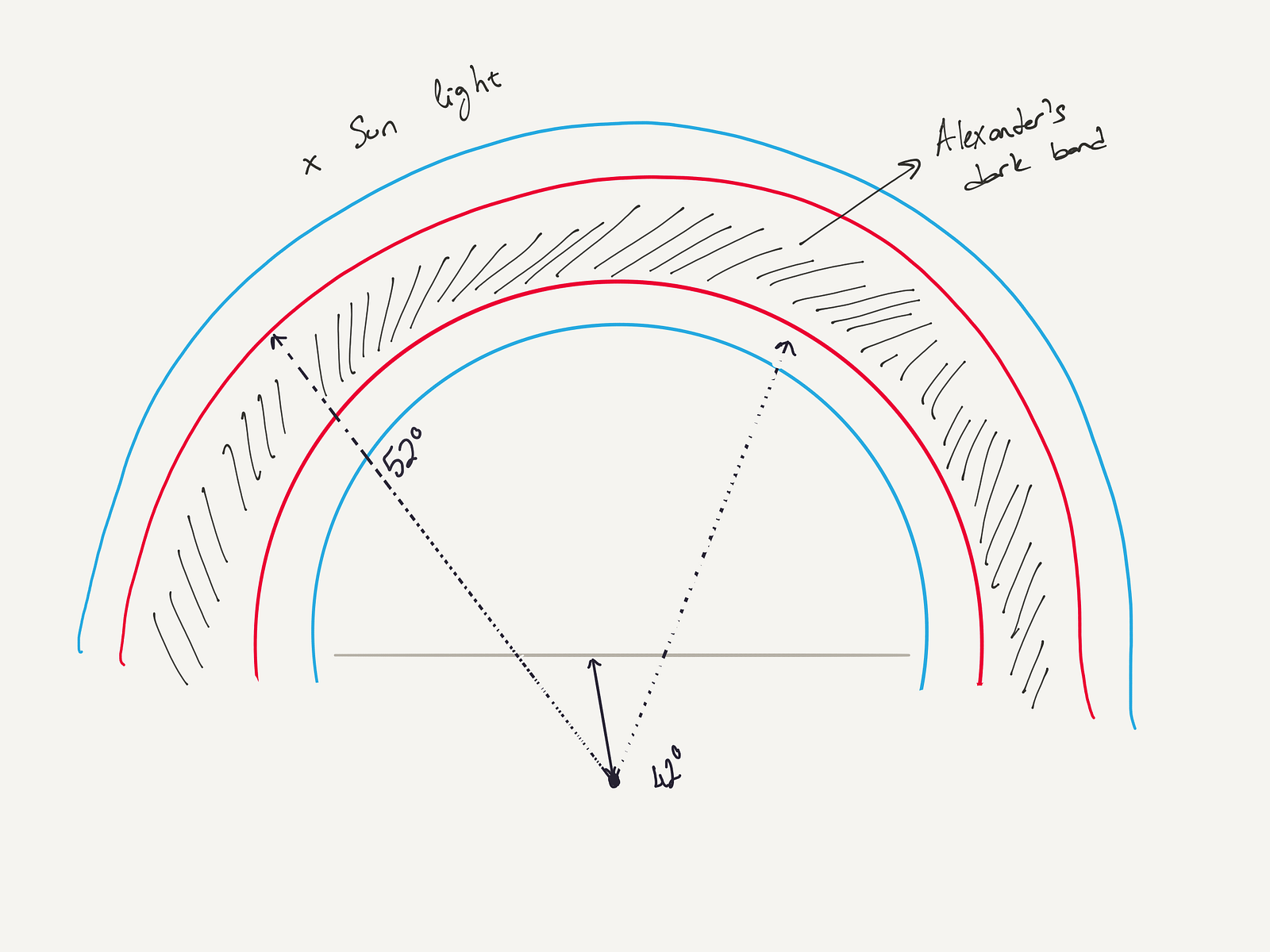Figure 11.2

### Some examples…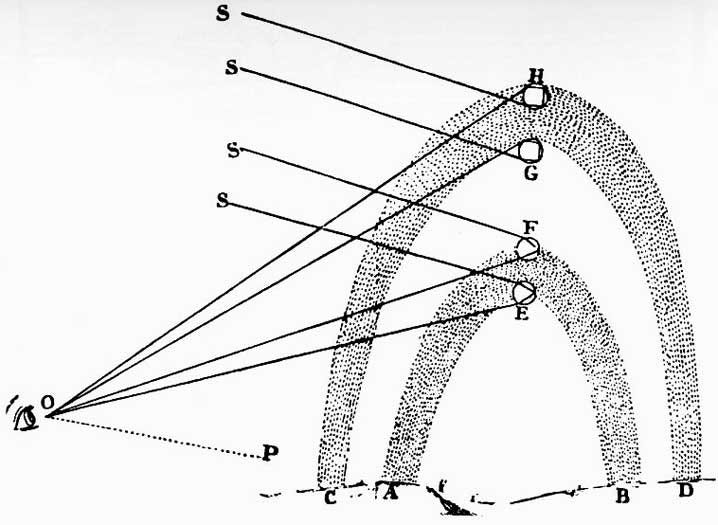Image 1: René Descartes’ sketch of how primary and secondary rainbows are formed (probable engraver: Frans van Schooten the younger). Image 2: Rainbow over Woolsthorpe Manor, Newton’s birthplace, 7 October 1979, photographed by Professor R. L. Bishop on Kodachrome 64 film, with an Olympus O M i camera and Olympus lens 28 mm f/3.5. Image 3: This is a diagram was drawn by the famous physicist Newton who was one of the first to understand the rainbow, from his book Optics.

When you water your garden when the sun is high in the sky, you can get a rainbow all the way around you. 42° away from the line is always red. If you turn around and look back, it is red.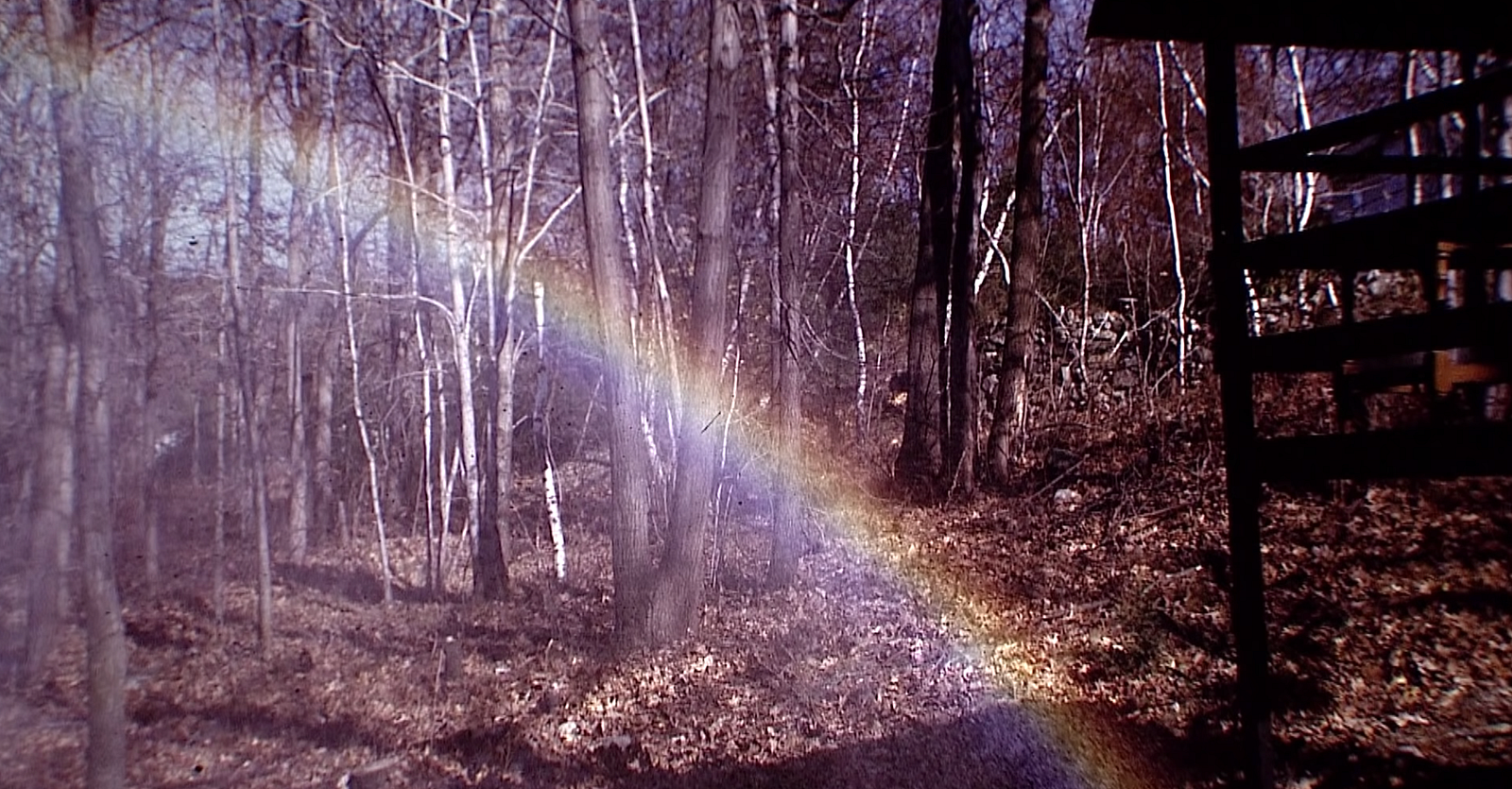Image 4: Walter Lewis’ backyard and his rainbow.

If you look at figures 5 and 6 carefully, the primary bow red is on the outside, and blue is on the inside. Alexander’s dark band can be seen in between the two bows. You cannot miss it. It shows up really dark. For the secondary bow, red is on the inside, and blue is on the outside.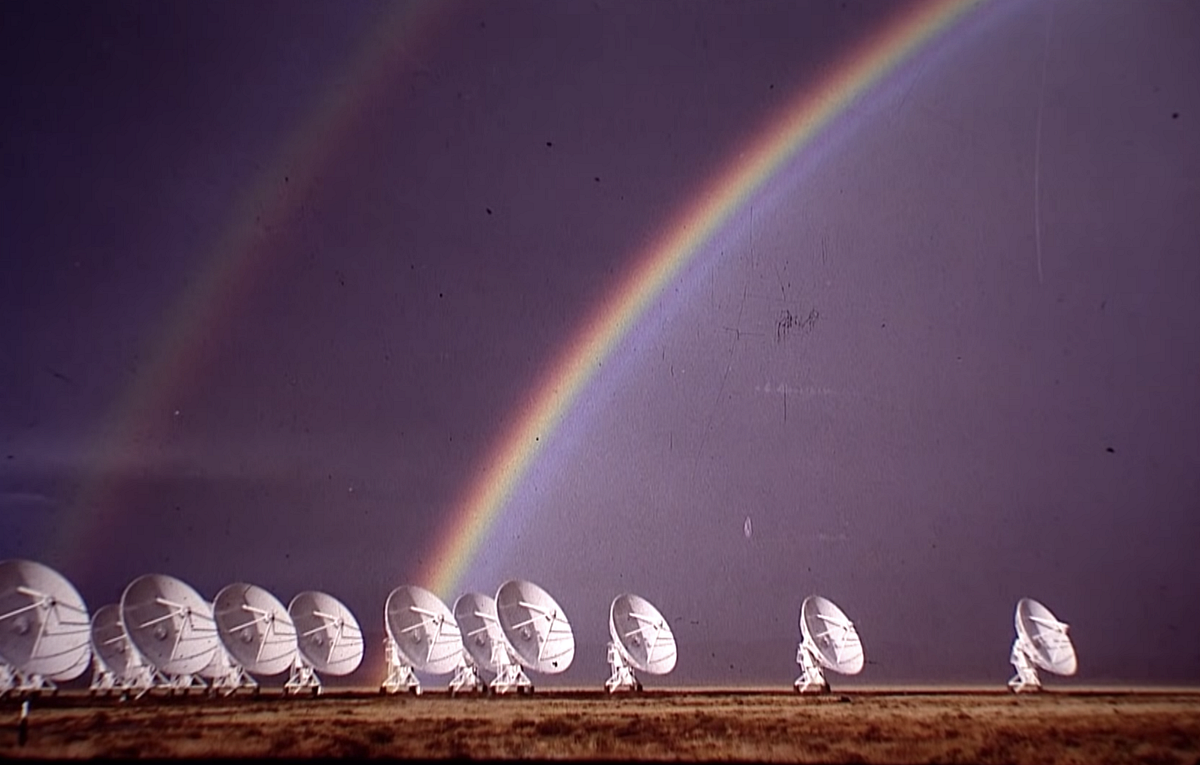Image 5 & 6 | Violet radio telescopes in Socorro New Mexico

Pictured is something called a white rainbow. It is very unique and rare. It is also called a fog bow, because fog has small water droplets that create this effect.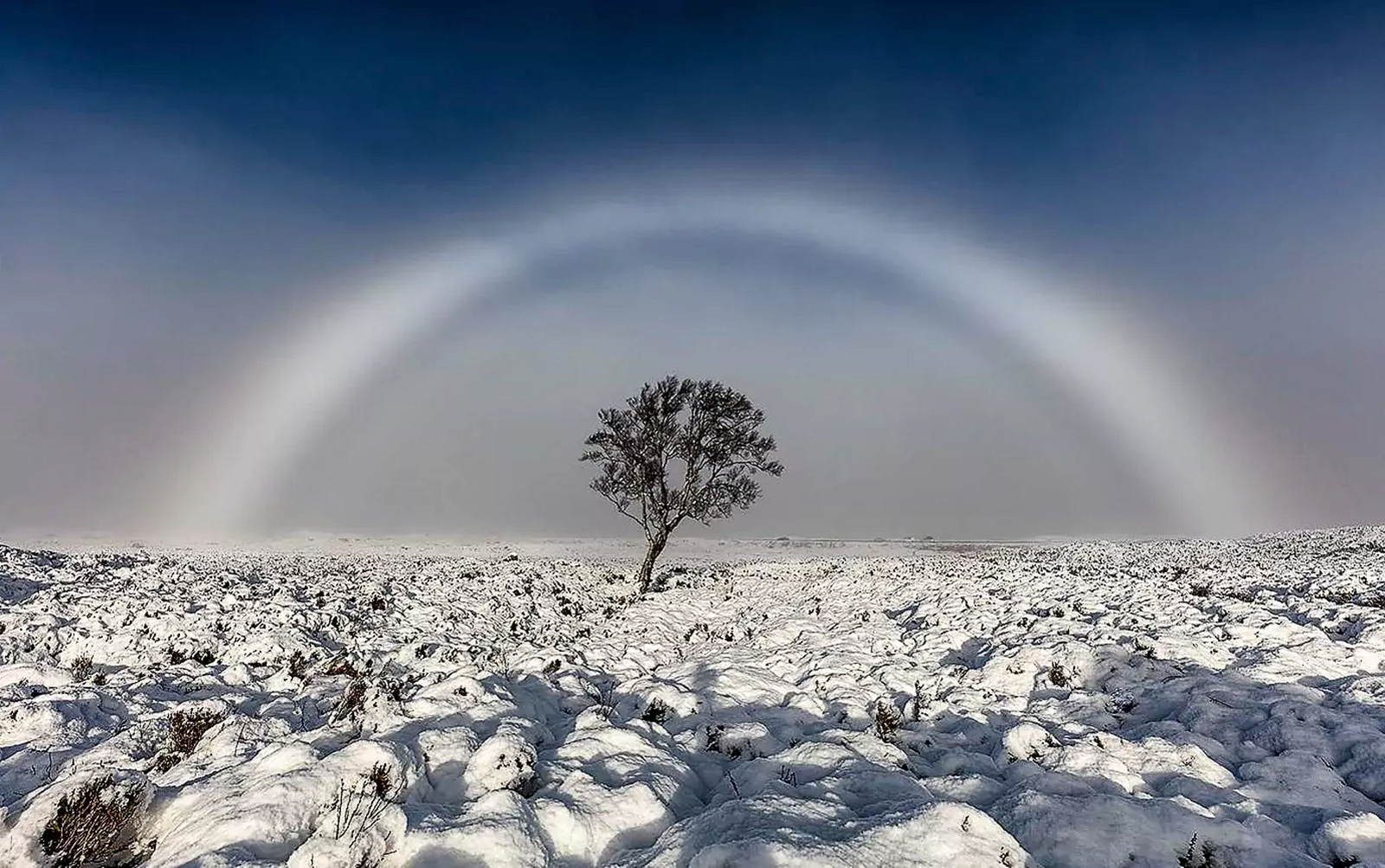Image 7 | White Rainbow

Let us imagine that there is a rainbow at sunset. What would you expect to see? We would only see red light because there is only red light. What would happen to the white light inside the bow? It would be red too.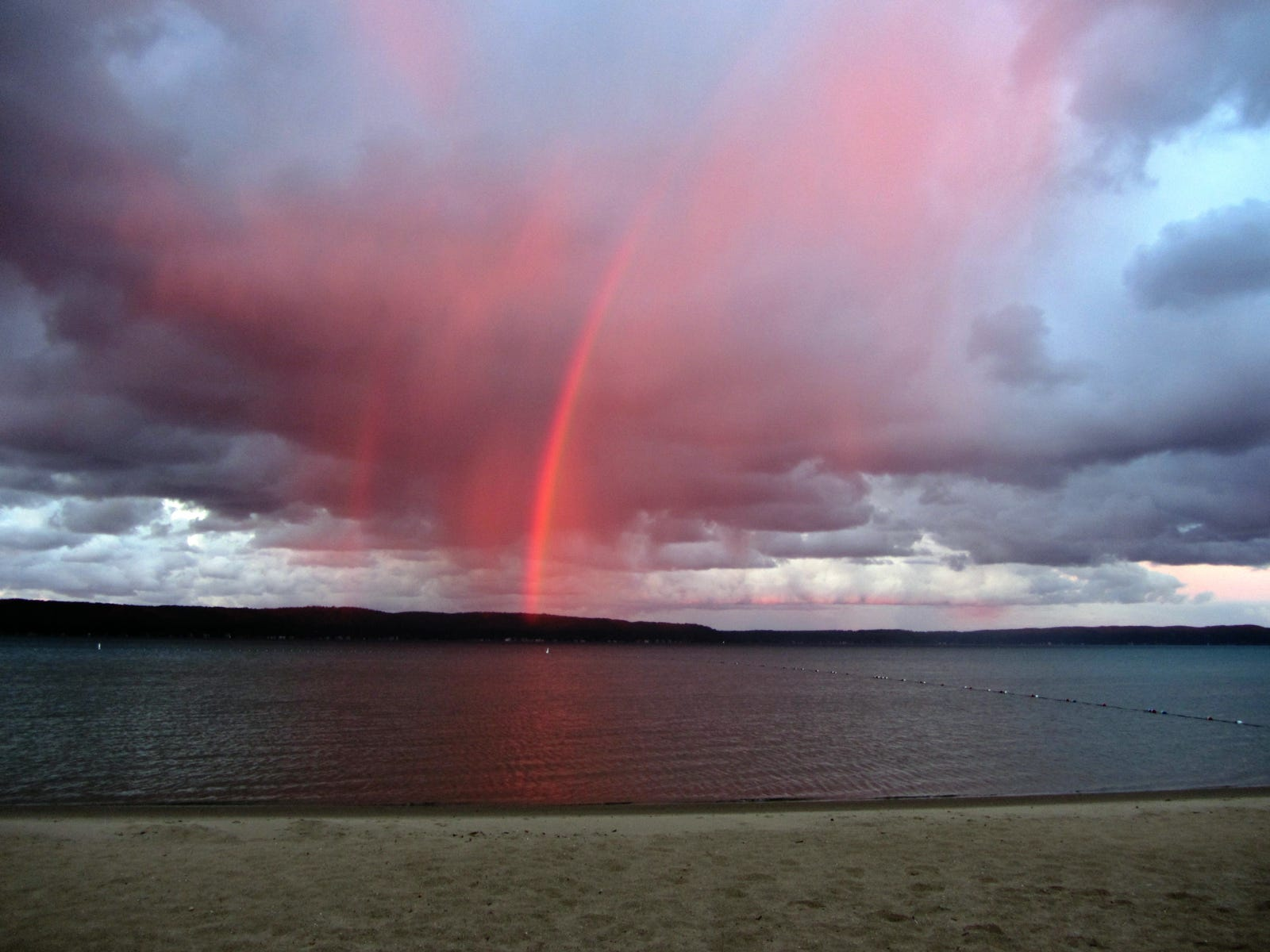Image 8 | Red rainbow.

I hope you are now able to truly see a rainbow when you look.